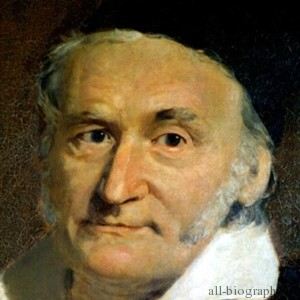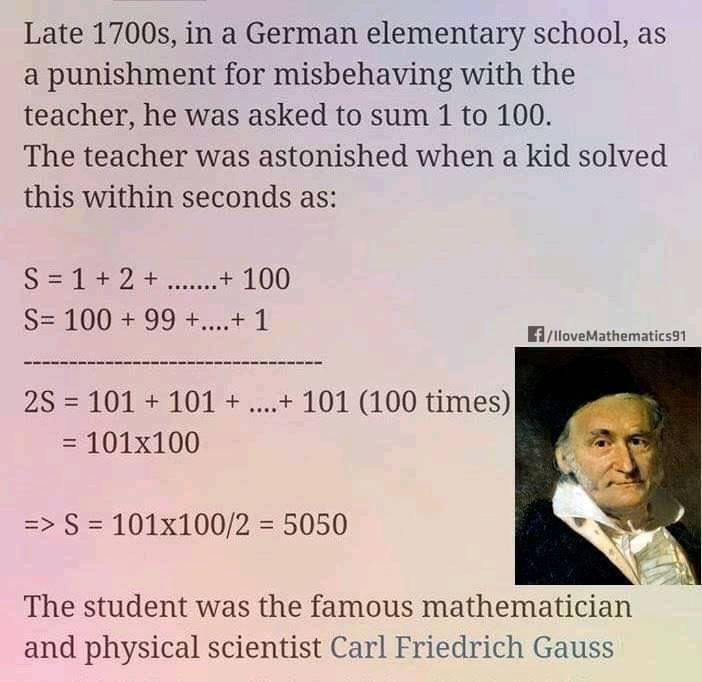# Carl Friederich Gauss"Mathematics is the queen of the sciences and arithmetic [number theory] is the queen of mathematics." [Carl Friedrich Gauss]

Johann Carl Friedrich Gauss; (Latin: Carolus Fridericus Gauss) (30 April 1777 - 23 February 1855) was a German mathematician and scientist who contributed significantly to many fields, including number theory, statistics, analysis, differential geometry, geodesy, geophysics, electrostatics, astronomy and optics. Sometimes known as the Princeps mathematicorum (Latin, "the Prince of Mathematicians" or "the foremost of mathematicians") and "greatest mathematician since antiquity", Gauss had a remarkable influence in many fields of mathematics and science and is ranked as one of history's most influential mathematicians. He referred to mathematics as "the queen of sciences." (from Wikipedia)

On this day Johann Carl Friedrich Gauss (1777-1855), the Prince of mathematicians, died. He was a German mathematician who transformed nearly all areas of mathematics, for which his talent showed from a very early age. For his contributions to theory in magnetism and electricity, a unit of magnetic field has been named the gauss. He devised the method of least squares in statistics, and his Gaussian error curve remains well-known. He anticipated the SI system in his proposal that physical units should be based on a few absolute units such as length, mass and time. In astronomy, he calculated the orbits of the small planets Ceres and Pallas by a new method. He invented the heliotrope for trigonometric determination of the Earth's shape. With Wilhelm Weber, he developed an electromagnetic telegraph and two magnetometers.